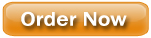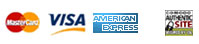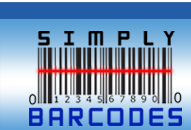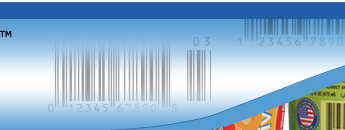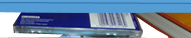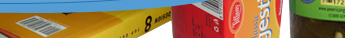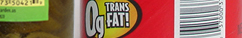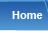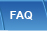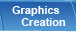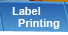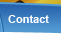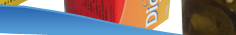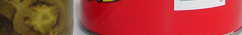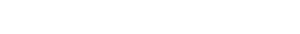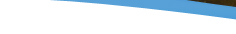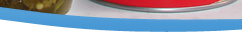5-step algorithm for check digit calculation:

Let's assume that we are using the fictitious code 05432122345.
1. Add all of the digits in the odd positions (digits in position 1, 3, 5, 7, 9, and 11)
0 + 4 + 2 + 2 + 3 + 5 = 16
2. Multiply by 3.
16 * 3 = 48
3. Add all of the digits in even positions (digits in position 2, 4, 6, 8 and 10).
5 + 3 + 1 + 2 + 4 = 15
4. Sum the results of steps 3 and 2.
48 + 15 = 63
5. Determine what number needs to be added to the result of step 4 in order to create a multiple of 10.
63 + 7 = 70
The check digit therefore equals 7.

UPC bar code check digit calculator
 Enter or paste 11 digit UPC

EAN bar code check digit calculator
 Enter or paste 12 digit code

SCC-14 bar code check digit calculator
 Enter or paste 13 digit code